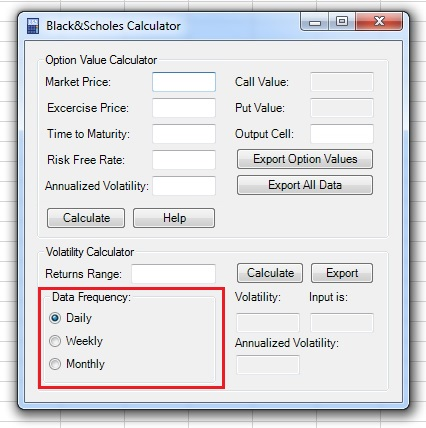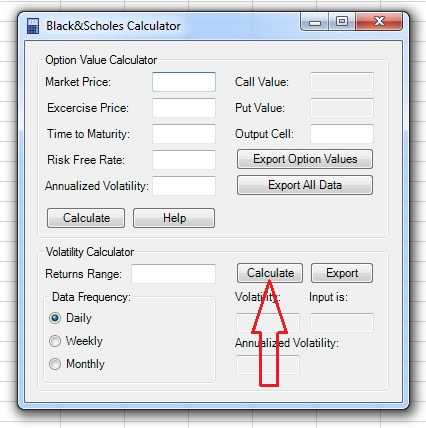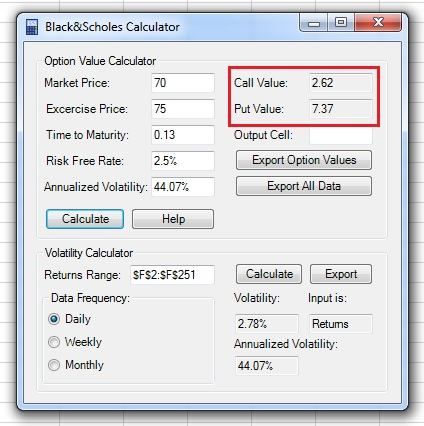This is the Black and Scholes Option Value Calculator tutorial.

You can watch the tutorial video, read the step by step text tutorial or go through the step by step screenshots tutorial

##### Step by Step Text Tutorial:

1. Click on the B&S Calc icon in the ADD-INS toolbar. The Black and Scholes Option Value Calculator window will open.

2. Input the main parameters:

• Market Price: current market price of the underlying security.
• Exercise Price: the exercise price of the option.
• Time to Maturity: the time to maturity of the option. May be expressed in years or as exercise date (in this case,  B&S Calculator will calculate years to maturity between current date and exercise date).
• Risk Free Rate: the risk free rate with the same maturity as the option.
• Annualized Volatility: the annualized volatility of the returns of the underlying security.

If you know the annualized volatility, skip action items 3 to 5 and continue from action item 6.

If you want to calculate the annualized volatility using data of the prices or returns of the underlying security, continue to action item 3:

3.  You can use historical data of prices or returns of the underlying security to calculate the annualized volatility.  Select the data range by clicking on the “Returns Range” field, selecting the range in the spreadsheet and returning to the Black and Scholes Option Value Calculator window. Note that you can select prices data or returns data from a single row or column.

Note: If the range input field is not empty, you need to delete existing text before selecting a new range in the spreadsheet.

4. Select the frequency of the selected data in the Data Frequency section: Daily, Weekly or Monthly.

5. Click on the “Calculate” button in the Volatility Calculator section and the volatility, annualized volatility and input type will appear in the appropriate fields. Make sure that Black and Scholes Option Value Calculator correctly identified input type (prices/returns). You can click on the “Export” button to export the annualized volatility to the Option Value Calculator section.

6. Click on the “Calculate” button in the Option Value Calculator section and the Call and Put values of the option will appear in the appropriate fields.

7. Select the first output cell by clicking on the “Output Cell” field, clicking on the cell in the spreadsheet and returning to the Black and Scholes Option Value Calculator window.

Note: If the range input field is not empty, you need to delete existing text before selecting a new range in the spreadsheet.

8. Click on the “Export Option Values” button to export only the values of the option, or on the “Export All Data” button to export the values and the data used as input in the calculation.

##### Step by Step Screenshots Tutorial:

1. Click on the B&S Calc icon in the ADD-INS toolbar. The Black and Scholes Option Value Calculator window will open:2. Input the main parameters:

• Market Price: current market price of the underlying security.
• Exercise Price: the exercise price of the option.
• Time to Maturity: the time to maturity of the option. May be expressed in years or as exercise date (in this case,  B&S Calculator will calculate years to maturity between current date and exercise date).
• Risk Free Rate: the risk free rate with the same maturity as the option.
• Annualized Volatility: the annualized volatility of the returns of the underlying security.If you know the annualized volatility, skip action items 3 to 5 and continue from action item 6.

If you want to calculate the annualized volatility using data of the prices or returns of the underlying security, continue to action item 3:

3.  You can use historical data of prices or returns of the underlying security to calculate the annualized volatility.  Select the data range by clicking on the “Returns Range” field, selecting the range in the spreadsheet and returning to the Black and Scholes Option Value Calculator window. Note that you can select prices data or returns data from a single row or column.

Note: If the range input field is not empty, you need to delete existing text before selecting a new range in the spreadsheet.4. Select the frequency of the selected data in the Data Frequency section: Daily, Weekly or Monthly.5. Click on the “Calculate” button in the Volatility Calculator section and the volatility, annualized volatility and input type will appear in the appropriate fields. Make sure that Black and Scholes Option Value Calculator correctly identified input type (prices/returns). You can click on the “Export” button to export the annualized volatility to the Option Value Calculator section.6. Click on the “Calculate” button in the Option Value Calculator section and the Call and Put values of the option will appear in the appropriate fields.7. Select the first output cell by clicking on the “Output Cell” field, clicking on the cell in the spreadsheet and returning to the Black and Scholes Option Value Calculator window.

Note: If the range input field is not empty, you need to delete existing text before selecting a new range in the spreadsheet.8. Click on the “Export Option Values” button to export only the values of the option, or on the “Export All Data” button to export the values and the data used as input in the calculation.